# Is your player better? Combining Bayesian statistics with Magnitude-based Inference.

Share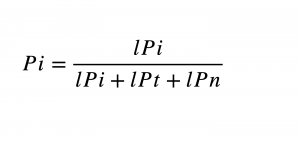Written by Peter Joffe.

After training, the player improved a little in the CMJ (counter-movement jump). Has he become more explosive in the game? Perhaps we have some hope, but still, we are not sure. First, the improvement itself is not that big. Secondly, CMJ does not provide a comprehensive description of the explosiveness in the game. What if we add a standing long jump? Hmm… he’s got a slight improvement. What about acceleration? 10 m sprint? Here we also have a minor improvement.

So each test individually remains a bit vague, but what if we could group them in some statistical analysis? Then, perhaps our conclusions will become more reliable?

### Preface.

In this article, I would like to suggest a relatively simple statistical framework for evaluating physical and physiological testing. It combines the fairly popular magnitude-based inference approach with Bayesian statistics.  I made online calculator that will help you with automatic calculations.

In scientific research, there is a need to prove something to the scientific community, so the design, including the statistical one, must be pretty strict. In addition, we mainly use group statistics in scientific studies because data of individual athletes may not be enough for robust conclusions.

We, practitioners, don’t need to publish, and every athlete is essential. Thus it is crucial to evaluate every sportsperson individually.

Another consideration is that the proposed method may not be of much help in seconds-metres-kilogram sports. In this case, the relationship between tests and performance is more straightforward and easier to measure. Often they even use the same units. The competition conditions are mostly standard, as are the tests. If a weightlifter improved in the bench press test, did he improve in the clean-and-jerk in competition? You can measure both and get an answer.

However there is never a perfect correlation between a test and a football game or a boxing match. The physical demands in these competitions are wide and varied. Sports games or boxing matches are inherently unpredictable, whereas tests should be standardised. The proposed method aims to link testing to actual performance in these events.

### Testing a quality that is not measurable.

When working with players, boxers, fencers, etc., we want to evaluate the progress in developing one or another physical quality that our athlete possesses, for example, how explosive he is.

However, it should be noted that such qualities are immeasurable.

We can say that a player is explosive, but we can’t give him a specific “explosive number”.

That can do the test.

However, one test, even a good one, which usually measures a very narrow aspect of quality, gives us only a rough idea of an athlete’s explosive power.

Thus, measuring the quality of interest not by one but by a group of relevant tests may make sense. It’s like firing a machine gun instead of a single shot with a pistol. There are more chances to hit the target and deal more cumulative damage (in our case, this means getting more information).

For example: After training, the player improved a little in CMJ. Did he become more explosive in the game? Perhaps we start to have some hope, but we are not sure. Firstly, improvement itself is not significant. Secondly, CMJ alone maybe not comprehensively describe game explosiveness. So, let’s add other tests connected with the leg’s power. Let’s do a standing long jump and 10 m sprint. Suppose here we have slight improvement also.

So what is our confidence in the improvement of explosiveness now?

Taking every test separately leaves us with a bit of uncertainty, but what if we can group them in some statistical analysis? Then, perhaps our conclusions will be more robust.

So, what kind of analysis?

### What to analyse?

What we should take for analysis is an interesting question. Amplitude of changes? That seems sensible. There is no big problem that one test may be in metres and another in seconds. We can normalise changes as Z-score.

Still, a pure quantitative description of change may not be informative. It does not include information on how reliable the test itself is and what difference is considered worthwhile. For example, change after training is 0.2 of standard deviation (Z-score). Can we conclude that it is really meaningful? Perhaps it is just natural fluctuation?

An analysis addresses such questions—magnitude-based Inference (MBI).

It calculates a probability of change to happen rather than simply computing its value. We will discuss this method later.

### Why not the average?

As for “how to analyse”, it seems that the most obvious solution is to take the average of changes in the group of tests.

Taking into account previous arguments we may also calculate average probability instead of average score.

However, in my view, the average has limited benefits for assessing chances of change in a certain quality.

Just think about it. In our example, you have three tests with moderate probability of improvement in each. The average of the three will be a number that cannot be higher than the highest chances in a single test. Hence the average will also be a small number.

Will you be less uncertain?

This approach may not eliminate the uncertainty. It just averages it.

### Using Bayesian approach.

The Bayesian analysis uses an “updating approach” when dealing with information coming from various sources.

For example, you are in a dark forest and hear a slight rustle in the bushes. You suspect someone is here, but you’re not sure. So you began to listen carefully, and the sound repeats! And then, for a moment, a light shadow flickers! So even though in all three occasions the signal was weak (the average value is small), now your confidence is relatively high.

Thus, in the Bayesian approach, if new information supports your beliefs, it adds and enhances your confidence. Each subsequent test, where the chances of improvement are  higher than probabilities of other  outcomes, reinforces your belief that this quality has improved. Therefor, the overall score may be higher than the highest score for the individual test (in fact, you even expect that).

For example, after the first test, you believe with 70% confidence that the athlete improved. However, two other tests gave you just 50% chances. Thus, if you take an average, your confidence goes down, and you end up with 57% chance for improvement. Bayesian statistics, however, may give as big as 87%! (Table 1).

Is it logical to use this method? I think that it’s. It’s similar to our shooting analogy: you have a big hit (70%), then a smaller hit (50%), and then another minor hit. Your accuracy (average score on a test) may not be all that great, but collectively you hit the mark a lot.

I should note that the Bayesian method quantifies your confidence in change and does not change itself! But of course, the two are related. The more significant the improvement, the more certain you are that it is real.

What probability do we consider large, and what small? It depends. Because in sports testing we have three possible outcomes—positive, trivial, and negative changes—the non-informative probability (such as your guess before the test) is the same for each outcome, 33.3%. This means that a probability after testing of 30-40% is not enough for a confident conclusion. It is too close to “by-chance” result. Essentially, the lowest probability that can support specific belief is around 50%, so it is at least equal to the sum of the probabilities of two other outcomes.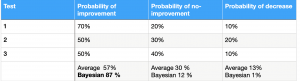Table 1. Bayesian analysis of three hypothetical tests.

Basically, you can stop reading here. If you find my idea reasonable and useful, Bayesian probability calculator  will automatically do the calculations for you. You need to enter three numbers for each test. One of them is the observed change (OC). It expresses a difference in the test before and after training.
The other two are typical test error (TE) and the smallest worthwhile change (SWC). You can find their values for particular test in the literature ( some examples in Table 2).

That is all.

However, it is better to understand things a little deeper and at least be able to calculate TE and SWC specifically for your athletes (see TE and SWC chapters).

Believe me, it’s not that difficult.

So let’s continue.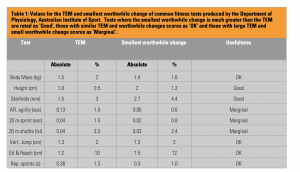Table 2. TE (TEM) and SWC  for some tests. Adopted from Pyne (2003).

### Updating your beliefs —Bayesian approach.

Suppose you tend to believe that your player has improved.

However, two other outcomes are possible: the player has not changed (a trivial change), and the player has, in fact, become worse.

You want to be unbiased, so you give the same odds to all three possible outcomes (33.3% chance). Thus, your initial knowledge is not informative. So, you decided to test the player.

Our task is to quantify the probability of improvement (Pi) given new information (test results).

To do this, you need to weigh the chances that your test results are compatible with improvement- likelihood of improvement (lPi)- against the sum of the likelihoods of all three outcomes.

I repeat that in our case, we have three hypotheses thus three likelihoods: lPi—improvement; trivial changes (lPt) and negative changes (lPn).

So general formula is:In its turn, the likelihood of hypothesis is a product of all sources of information, in our case, from all tests:

Likelihood (positive)= Likelihood (positive) test1 x Likelihood (positive) test2 x Likelihood (positive) test3…. The same procedure should be applied to other outcomes.

Example:

If we made two tests and the likelihood of a positive result is 0.7 for the first and 0.5 for the second, then the overall likelihood of improvement is:

lPi=0.7 x 0.5=0.35.

Please note that this likelihood is not a final probability of improvement. We still need to divide it by the sum of all likelihoods.

We will talk about how to find likelihoods in a test later. But, for now, let’s take a simple example:

We tested players in two tests—countermovement jump (CMJ) and standing long jump (SLJ)— at the beginning and end of pre-season. We want to quantify the probability of overall improvement. The CMJ likelihoods of positive, trivial, and negative changes were 0.7, 0.2 and 0.1, respectively. In SLJ these were 0.6; 0.3 and 0.1.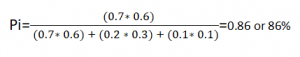You can see that our confidence in improvement became higher after two tests than it might be after one.

### Finding likelihoods: Magnitude-based inference.

MBI is a statistical method that has recently become quite popular in sports science.

However, this causes controversy in the scientific community and criticism from statisticians. I can’t judge how appropriate this method is for group statistics in research papers, but it seems plausible to me when you’re evaluating a person.

For the presented idea, the primary purpose of MBI is to find likelihoods for further Bayesian analysis.

Three inputs are required for MBI analysis, and I have mentioned them before: OC, TE and SWC.

### Typical error.

If you could test an athlete an infinite number of times and then take the average, that would be the “true” value. However, it is impossible to do this in reality. Thus, we assume that the result observed in the test is always different from the hypothetical true result. This difference is measurement error or typical error (TE). This error may occur due to random changes in test conditions and procedure. So, how can we find TE if we never know the true value? I will describe two relatively simple ways that suit the practitioner.

Two ways of calculating TE.

First, if you have this option, test your athlete 8-10 times in a short period.

There are several necessary conditions. First, the time between tests should be long enough to prevent fatigue and short enough to avoid changes in fitness. The test should be as standard as possible (testing equipment, time of day, fatigue state, diet, etc.). In addition, it would be better if the test was simple and familiar to the athlete to rule out improvement simply by learning. After all, we want to make sure that these differences are natural and random fluctuations in the testing process, and not training and learning. If these conditions are met, then the test results’ standard deviation (SD) is equal to TE.

For example, you tested athlete A in countermovement jump ten times and got following results (cm):

47; 49; 48; 46; 49; 47; 50; 49; 48; 50

Then SD (and TE) will be 1.27  (Online SD calculator).

The second way. If you have a homogeneous group of athletes, test them twice (same conditions apply for timing between tests), calculate the SD of individual changes and divide by 1.4 (√2) (for a detailed explanation of where √2 came from, see article). This method is better for excluding learning and perhaps easier than testing the same athlete multiple times.

Just make sure it’s not the SD of the results like in the first case — it’s the SD of the changes! For instance, you tested ten athletes (A;B;C;D;E;F;G;H;I;J) two times and calculated difference between two tests for each athletes. Let’s say you got (Test 2 minus Test1):

A: -1    B:  3    C:  -2    D:  2     E:  -1    F: -2    G: 3    H: -1    I:  -1   J:  0

Then   SD=1.84;  TE=1.84/√2=1.31

### How many participants or trials do you need?

Regarding how many tests (option 1) or athletes (option 2) you need, it can be said that the effect of this number is more pronounced at the extreme ends of the distribution.

That means that the high and low probabilities depend more on the number of tests/participants than the mid-range probabilities.

For example:

The 10-participant test gives odds of 9.6% at the lowest extreme (t-value 1.41) compared to a “perfect” normal distribution of 8% (-1.41 Z).

In the middle range (closer to 50%), the same number of participants (t-value 0.471) gives 32%, which is the same as for the normal distribution (-0.471 Z).

So I think 8-10 tests/participants will be enough for our purposes.

In the case of 5-7 tests/participants, you will possibly need a little more complex analysis (T-distribution) and get more considerable uncertainty (especially at extremes).

Less than 5? Perhaps it is not enough.

### SWC.

Another variable for MBI analysis is SWC. That is the smallest training-related change in the test  that we consider meaningful.

For statistical analysis SWC is important because it defines the relationship between real change and “noise” in the test.

When calculating likelihood (probabilities), we consider the interval of change 0 ± SWC as “No change” or trivial (Picture 1).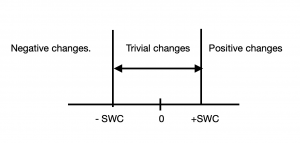Picture 1. SWC.

Thus, if an athlete has reached 50 cm in CMJ and we take SWC as 1 cm, then future results between 49-51 cm will be considered as a negligible change.

Therefore, if we make SWC too conservative, then the area of trivial changes may be too big, and we will miss real changes.

Make it too small, and you might confuse the changes with noise (TE).

In sports performance, which is already subject to variation and uncertainty, the choice of subjective SWC can be confusing.

Therefore, there is a suggestion to standardise SWC by connecting it to SD or CV (Coefficient of Variation).

So SWC can be:

0.3 CV in track and field events.

0.2 between-subjects SD in team sports.

I suggest making SWC equal to TE in physical testing.

In this case, all changes “covered” by noise are considered trivial.

It leaves less room for ambiguity and helps to avoid overoptimistic conclusions (as it may happen when SWC is less than TE).

### Combining MBI with Bayesian stats.

Now we have all that is needed for the final analysis

I will give an example of by-hand calculation in the appendix.

For those who are not interested or have no time — it’s ok.

As I said, the Magnitude-based Bayesian calculator makes calculations automatically.

You need to enter three variables OC , TE and SWC (calculated as described above or taken from literature) and you will get the probabilities for positive, trivial and  negative  outcomes.

### When can we use this approach?

Endurance tests can be quite unreliable for finding TE with sufficient accuracy. In addition, these tests require high motivation and are difficult to repeat in a short time.

Submaximal testing may be more appropriate for suggested analysis. For example, it is always a problem to access player preparedness and current form. No individual test can give a reliable answer. Perhaps, we can combine HR, RPE, and HR-recovery during a submaximal run to address this problem.

I think in  physiological testing, the combination of VO2max, blood lactate and respiratory thresholds might also be interesting. All of them are related to aerobic endurance, and together they can be better than either of them individually.

As discussed earlier, Bayesian-MBI analysis can help assess strength, power, and speed associated with performance.

It is important to emphasise that in any case, all tests should be aimed at the same target – measuring the same quality.

### Conclusion.

The main advantage of the proposed method is the combination of Bayesian statistics with MBI, which allows a more comprehensive assessment of changes in a specific performance-related quality.

It combines different tests with different units, TEs and SWCs and gives the coach an overall picture of changes that can be transferred to performance improvement.

Moreover, the Bayesian approach allows for a “continuously informative approach” where the results of previous testing can be considered “prior” for new analysis.

However, it is essential that the tests in the analysis match the quality in question; otherwise, Bayesian stats will be inappropriate! Again, the shooting analogy: all tests must be aimed at the same target.

In my opinion, the suggested idea and math behind it are relatively simple.

However, I should note that the main limitation of mathematics in human performance is not the mathematical apparatus per se but rather the data that you feed into it.

We work with people, so the environment is quite changeable, unpredictable and subject to multifactorial influences. Thus, the collected data can be pretty unreliable. That can make further mathematical analysis unreliable also.

However, in my opinion, the proposed method can help  to reduce the uncertainty.

### Appendix.

Example:

Athlete made three tests: CMJ, SLJ and 10 m acceleration (10) pre and post training.

We have OCs; TEs and SWCs:

Test1 (CMJ):  OC= 3 cm  SWC=1cm TE=1cm

Test 2 (SLJ):    OC=5 cm SWC=3 cm TE=3cm

Test 3  (10):  OC=0.1 sec  SWC=0.1 sec TE=0.1 sec

We need to calculate:

1. SD of changes= TE x 1.4 (√2)

2.  Distance between points -SWC and OC normalised for SD: ( -SWC -OC) / SD. That is input for Z table which gives us probability of harmful changes

3. In the same manner we should calculate distance from +SWC to OC: (+SWC-OC)/SD That is Z-table input which gives probability for harmful+trivial changes

4. Probability for harmful+trivial changes (step 3) minus harmful changes ( step 2)=probability of trivial changes.

5. 1- probability of harmful changes + trivial changes (step 3)= Probability of positive changes.

After calculating all probabilities (likelihoods) for all tests we  may perform Bayesian analysis: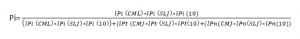Let’s enter numbers:

Test 1 (Picture 2):

SD=1×1.4=1.4

Distance from point -SWC  to  OC= -1-3=-4

This is -4/1.4=-2.86 SD

Using Z table for -2.86: negative changes probability= 0.0021

Distance from point +1 SWC to OC is: 1-3= -2

That is -2/1.4=-1.43 SD

Z table for -1.43 = 0.0764

0.0764-0.0021=0.0743 probability of trivial changes

1-0.0764=0.9236 probability of positive changes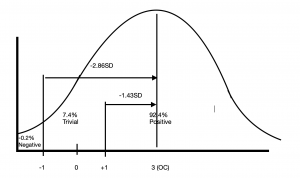Picture 2. Test 1. Magnitude-based analysis.

Test 2 (Picture 3) :

SD= 3×1.4=4.2

Distance -1SWC= -3-5=-8

-8/4.2=-1.90SD

Z table gives 0.0287 probability negative changes

Distance +SWC=3-5=-2

-2/4.2=-0.48 SD

Z table: 0.3156

0.3156-0.0287=0.2869 Trivial changes

1-0.3156=0.6844 Postive changes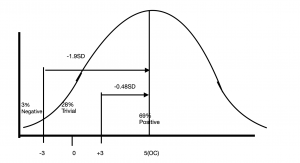Picture 3. Test 2.

Test 3 :

SD= 0.1 x 1.4= 0.14

Distance -SWC= -0.1-0.1= -0.2

-0.2/0.14= -1.43 SD

Z table = 0.0764 negative changes

Distance +SWC = 0.1- 0.1=0

above 0 SD   Z-table= 0.5

0.5 – 0.0764=0.4236 trivial changes.

Positive changes=0.5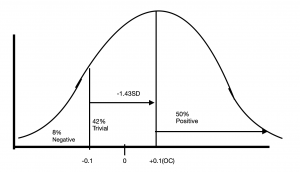Picture 4. Test3.

Overall Bayesian calculation: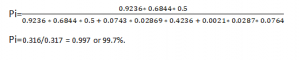### References.

1. Batterham, A. M., & Hopkins, W. G. (2006). Making meaningful inferences about magnitudes. International Journal of Sports Physiology and Performance, 1(1), 50-57.

2. Buchheit, M. (2016). The Numbers Will Love You Back in Return-I Promise. Int J Sports Physiol Perform, 11(4), 551-554.

3. Interpreting the result of fitness testing (Pyne, 2018).

4. Hopkins, W. G. (2000). Measures of reliability in sports medicine and science. Sports Medicine, 30(1), 1-15.

5. Magnitudes matter more than beetroot juice https://sportperfsci.com/magnitudes-matter-more-than-beetroot-juice/

6.Pyne, David B. “Interpreting the results of fitness testing.” In International science and football symposium, pp. 1-6. Victorian Institute of Sport Melbourne, Australia, 2003.

7.. Swinton, Paul A., Ben Stephens Hemingway, Bryan Saunders, Bruno Gualano, and Eimear Dolan. “A statistical framework to interpret individual response to intervention: paving the way for personalized nutrition and exercise prescription.” Frontiers in Nutrition 5 (2018): 41.

8. Want to see my report coach? https://martin-buchheit.net/2017/02/18/want-to-see-my-report-coach/

9. Weir, Joseph P. “Quantifying test-retest reliability using the intraclass correlation coefficient and the SEM.” The Journal of Strength & Conditioning Research 19, no. 1 (2005): 231-240.

.

Share